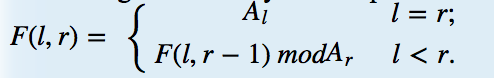## 描述

The shorter, the simpler. With this problem, you should be convinced of this truth.

You are given an array A of N postive integers, and M queries in the form (l,r). A function F(l,r) (1≤l≤r≤N) is defined as:You job is to calculate F(l,r), for each query (l,r).

### 输入

There are multiple test cases.

The first line of input contains a integer $T$, indicating number of test cases, and $T$ test cases follow.

For each test case, the first line contains an integer $N(1≤N≤100000)$.
The second line contains $N$ space-separated positive integers: $A_1,…,A_N$ $(0≤A_i≤10^9)$.
The third line contains an integer $M$ denoting the number of queries.
The following $M$ lines each contain two integers $l,r (1≤l≤r≤N)$, representing a query.

### 输出

For each query$(l,r)$, output $F(l,r)$ on one line.

1
3
2 3 3
1
1 3

2